### 3.2. Scattering of photons by an electron population

The distribution of photon frequency shifts caused by scattering by a population of electrons is calculated from P (s;) by averaging over the electrondistribution. Thus for photons that have been scattered once, the probability distribution of s, P1 (s), is given by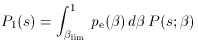(33)

wherelim is the minimum value ofcapable of causing a frequency shift s,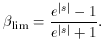(34)

The limitations of equation (33) are evident from the assumptions made to derive equation (28). That is, the electron distribution pe() must not extend to sufficiently large Lorentz factors,, that the assumptions of elastic scattering with the Thomson scattering cross-section are violated. For photons of the microwave background radiation these assumptions are amply satisfied provided that2 x 109. In clusters of galaxies the typical electron temperatures may be as much as 15 keV (1.8 x 108 K), but the corresponding Lorentz factors are still small, so that we may ignore relativistic corrections to the scattering cross-section.

If the electron velocities are assumed to follow a relativistic Maxwellian distribution,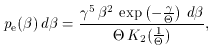(35)

whereis the dimensionless electron temperature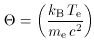(36)

and K2(z) is a modified Bessel function of the second kind and second order, then the resulting distribution of photon frequency shift factors can be calculated by a numerical integration of equation (33).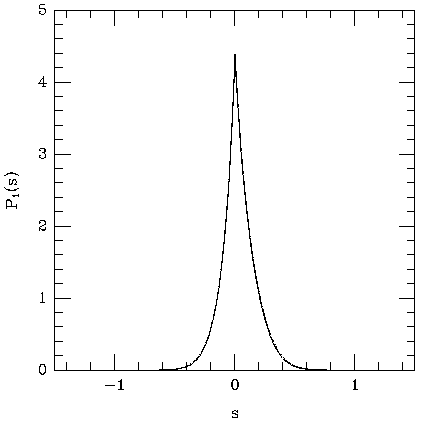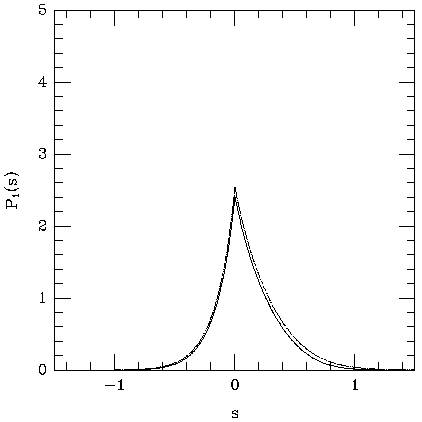Figure 5. The scattering kernel, P1 (s), for gases at 5.1 keV and 15.3 keV. The solid line shows the scattering kernels calculated according to (33), as derived by Rephaeli (1995a). The dotted line indicates the scattering kernels as calculated by Sunyaev (1980), based on the results of Babuel-Peyrissac & Rouvillois (1969).

The result of performing this calculation for kB Te = 5.1 and 15.3 keV is shown in Fig. 5, where it is compared with the result given by Sunyaev (1980). It can be seen that the distribution of scattered photon frequencies is significantly asymmetric, with a stronger upscattering (s > 1) tail than a downscattering tail. This is the origin of the mean frequency increase caused by scatterings. As the temperature of the electron distribution increases, this upscattering tail increases in strength and extent. Sunyaev's (1980) distribution function tends to have a stronger tail at large values of s and a larger amplitude near s = 0 than does the form derived using (33).

It is also of interest to calculate the form of P1 (s) for a power-law distribution of electron energies in some range of Lorentz factors1 to2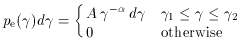(37)

with normalizing constant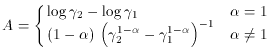(38)

since such a population, which might be found in a radio galaxy lobe, can also produce a Sunyaev-Zel'dovich effect. Synchrotron emission from radio galaxies has a range of spectral indices, but values of2.5 are common. Thus Fig. 6 shows the result of a calculation for an electron population with= 2.5. As might be expected, the upscattering tail is much more prominent in Fig. 6 than in Fig. 5, since there are more electrons with>> 1 in distribution (37) than in distribution (35) for the values ofandchosen.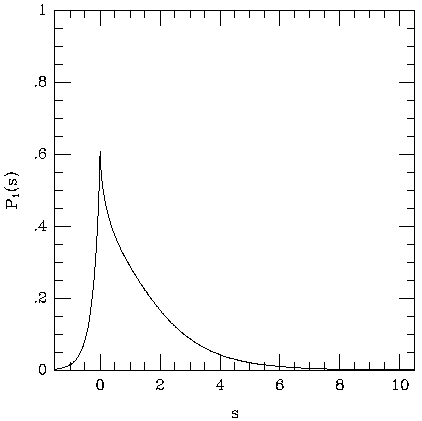Figure 6. The scattering kernel, P1 (s), for a power-law electron distribution with energy index= 2.5 (see equation 37). The stronger upscattering tail here, relative to Fig. 5, is caused by the higher proportion of fast electrons in distribution (37) than (35).